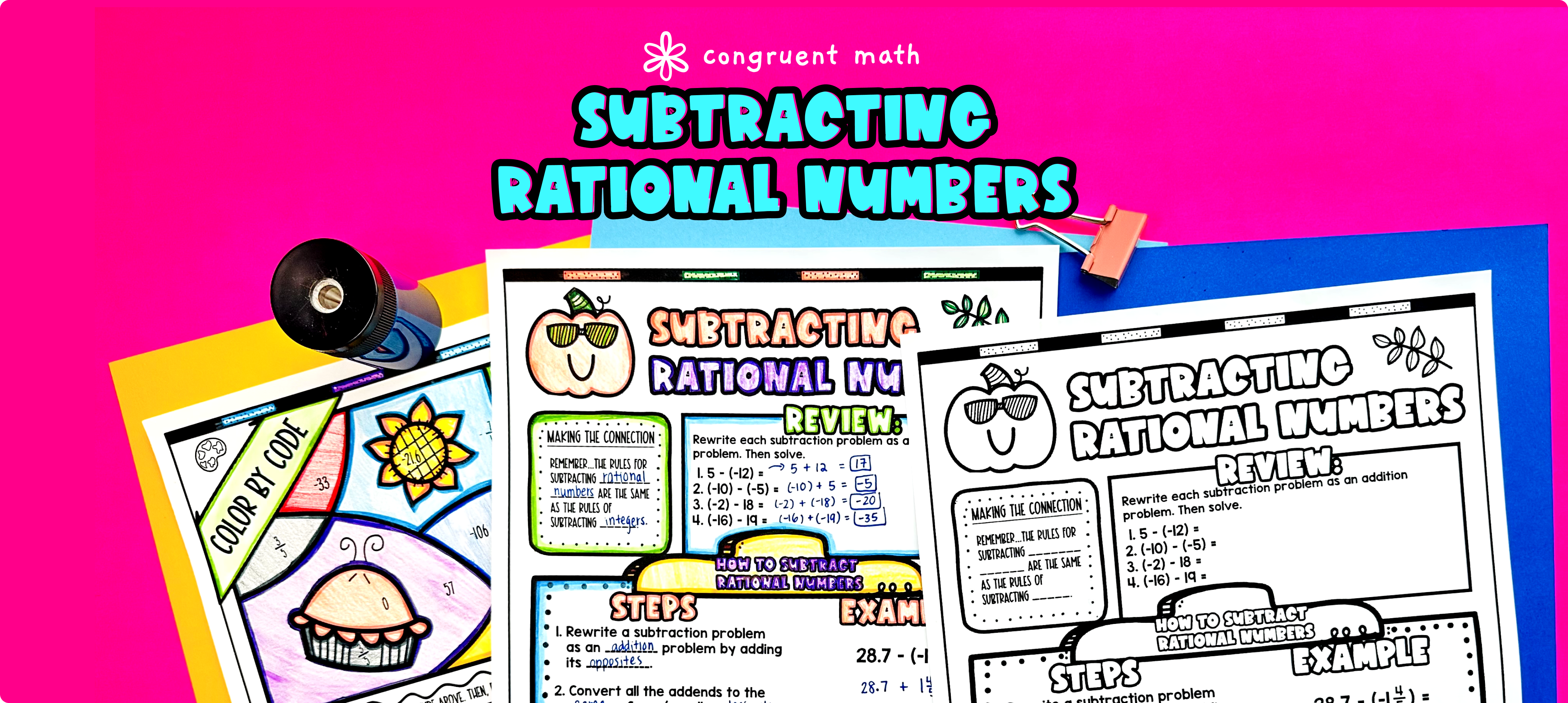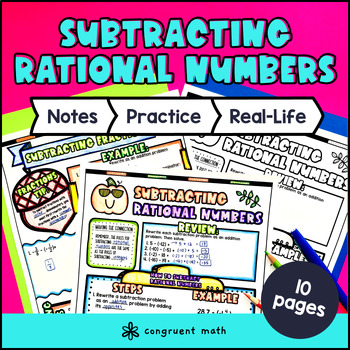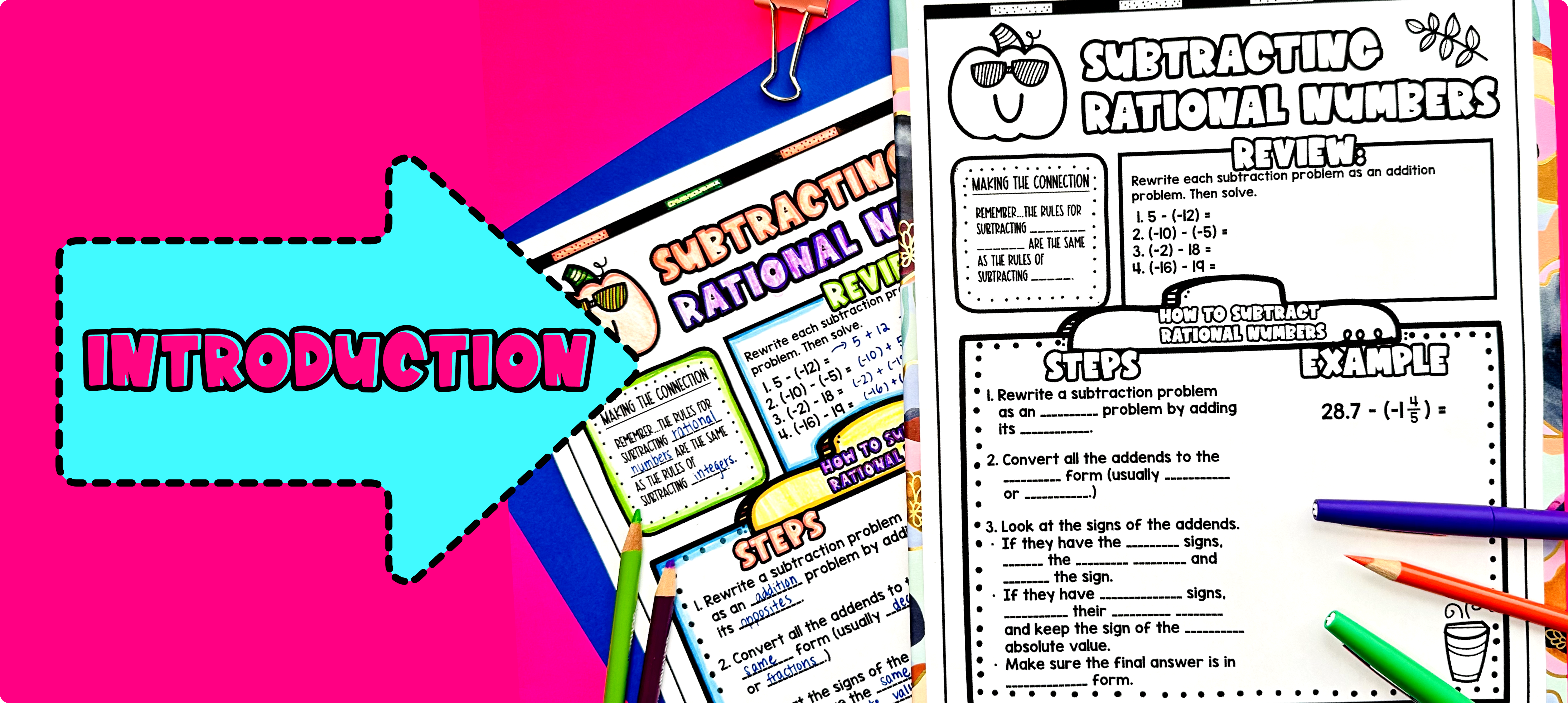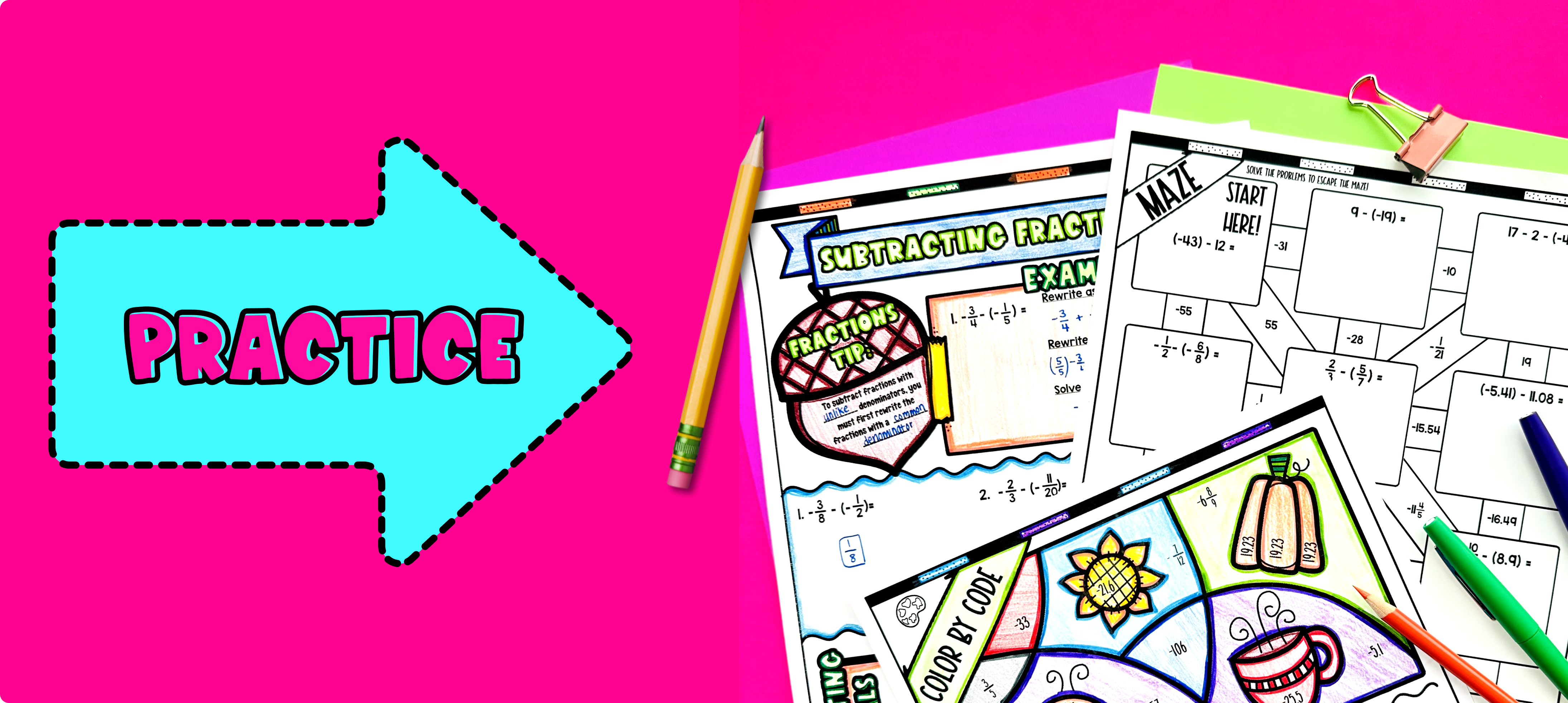# Subtracting Rational Numbers Lesson PlanPin This

## Overview

Ever wondered how to teach subtracting rational numbers in an engaging way to your 7th grade students?

In this lesson plan, students will learn about subtracting rational numbers and their real-life applications. Through artistic, interactive guided notes, check for understanding, a maze worksheet, and a color by number activity, students will gain a comprehensive understanding of subtracting rational numbers.

The lesson culminates with a real-life example that explores how to use these skills for online shopping and navigating discounts.

## Get the Lesson Materials\$4.25

## Learning Objectives

After this lesson, students will be able to:

• Subtract rational numbers including negative fractions, decimals, and integers

• Convert between different forms of rational numbers

• Apply the concept of subtracting rational numbers to real-life situations, such as online shopping and navigating discounts.

## Prerequisites

Before this lesson, students should be familiar with:

• Basic addition and subtraction for positive numbers

• Understanding of the concepts of fractions, decimals, and integers

• Convert between mixed fractions and improper fractions

## Key Vocabulary

• Rational numbers

• Fractions

• Integers

• Decimals

• Subtract

• Negative numbers

• Common denominators

• Opposites

• Absolute value

## Procedure

### IntroductionPin This

As a hook, ask students why understanding how to subtract rational numbers is important in real life, such as when shopping online or calculating discounts. Refer to the last page of the guided notes as well as the FAQs below for ideas.

Use the guided notes to introduce the concept of subtracting rational numbers. Walk through the key points of the topic of the guided notes to teach. Refer to the FAQ below for a walk-through on this, as well as ideas on how to respond to common student questions. If there’s a check for understanding integrated into the guided notes, have students walk through the “You Try!” section. Call on students to talk through their answers, potentially on the whiteboard or projector. Based on student responses, reteach concepts that students need extra help with.

### PracticePin This

There are 3 practice sheets included in the guided notes. First, have students practice subtracting rational numbers using the practice sheet (pg. 2). You can work on this together as the class.

Fast finishers can dive into the maze and color by number activities for extra practice. You can assign it as homework for the remainder of the class. Walk around to answer student questions.

### Real-Life ApplicationPin This

Bring the class back together, and introduce the concept of applying subtracting rational numbers to real-life situations, such as online shopping and navigating discounts. Discuss with the class how these skills can be used to find the best deals and save money. You can refer to the FAQs for more ideas on how to teach this topic. Have students work in pairs or small groups to find examples of online shopping or discounts that use subtracting rational numbers, and share their findings with the class. Encourage students to think critically about how they can use these skills in their own lives.

## Extensions

If you're looking for digital practice for adding and subtracting rational numbers, try these Pixel Art activities in Google Sheets. Every answer is automatically checked, and correct answers unlock parts of a mystery picture. It's incredibly fun, and a powerful tool for differentiation.

Here are 2 activities to explore: